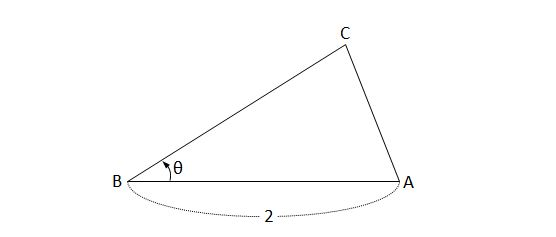# Rate of change of the area of a triangleIn triangle $\text{ABC},$ $\overline{\text{AB}}=2$ and $\overline{\text{BC}}+\overline{\text{CA}}=2\overline{\text{AB}}.$ Let $\angle \text{B}=\theta$ and suppose that the rate of change of the angle $\theta$ with respect to time is $\frac{d\theta}{dt}=2\ (\text{rad/sec}).$

Then, if $S$ is the area of the triangle, what is the rate of change of $S$ with respect to time when $\angle \text{A}=\frac{\pi}{2}?$

×